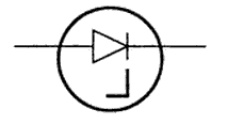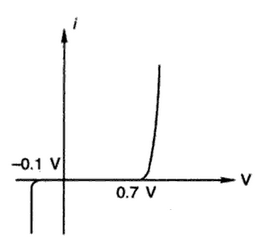### Backward Diodes

Zener diodes are normally used under the reverse bias condition. Their breakdown voltages are normally greater than 2 V. Forward conduction occurs in the voltage around 0.7 V (Si). The breakdown, i.e. Zener effect under the reverse bias condition can also be obtained near zero (around 0.1 V).
Figure 1 shows the symbol of backward diodeFig. 1 Symbol of backward diode

Figure 2 shows the characteristics of a backward diode. It is called a backward diode because it conducts better in the reverse than in the forward direction.Fig. 2 characteristics o f a backward diode

Figure 3 shows the low level rectifier circuit using a backward diode. Consider a sine wave with a peak o f 0.5 V is given as the input to the backward diode. This voltage (0.5 V) is not enough to forward bias to diode into conduction, but it is enough to breakdown the diode. Hence, the output is a rectified half wave signal with a peak of 0.4 V (0.1 V is lost across the diode). Backward diodes are used to rectify weak signals whose peak amplitudes are between 0.1 and 0.7 V.Fig. 3 low level rectifier circuit using a backward diode